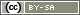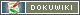[[random()]]

# random()

`int random(int min, int max)`

The function `random()` returns a whole number between min and max. It is suitable for random draws with replacement. Examples of its use as part of a random draw can be found in the chapter Randomization.

• min
The smallest number allowed
• max
The largest number allowed

Note: Although one could create an experimental randomization via `random()`, we strongly recommend using a Random Generator. For more information about randomization an experimental groups see Randomization.

Warning: The `random()` function does not store the random number drawn in the data set. If you need the drawn number, please use a Random Generator or store the function in an internal variable using `put()`.

## Return Value

A whole number between min and max

min ≤ return value ≤ max